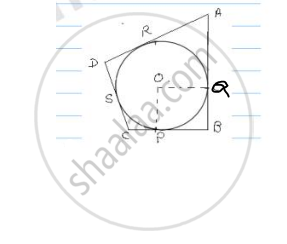Share

# In the Fig. a Circle is Inscribed in a Quadrilateral Abcd in Which ∠B = 90° If Ad = 23cm, Ab = 29cm and Ds = 5cm, Find the Radius of the Circle. - CBSE Class 10 - Mathematics

ConceptCircles Examples and Solutions

#### Question

In the fig. a circle is inscribed in a quadrilateral ABCD in which ∠B = 90° if AD = 23cm,
AB = 29cm and DS = 5cm, find the radius of the circle.

#### Solution

AB = 29 cm

∠B = 90°

DS = 5cm∠OPB = ∠OQB = 90° = ∠B = ∠POQ

and PO = OQ. ∴ POQB is a square PB = BQ = r

We know that

Tangents drawn from external point to circle are equal in length.

We know that

Tangents drawn from external point to circle are equal in length.

From A, AR = AQ …. (i)

From B, PB = QB …. (ii)

From C, PC = CS …. (iii)

From D, DR = DS …. (iv)

(i) + (ii) + (iv) ⇒ AR + DB + DR = AQ + QB + DS

⇒ (AR + DR) + r = (AQ + QB) + DS

AD + r = AB + DS

⇒ 23 + r = 29 + 5

⇒ r = 34 – 23 = 11 cm